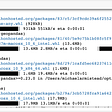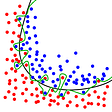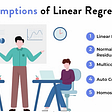# Regression

In regression we fit a line through the data points to predict a continuous target variable using various independent explanatory variables
Let the line be y= α+βx
We use the least squared error approach We square the error because:

1. It makes all errors positive and eliminates nullification of positive and negative error
2. It diminishes the errors which are less than one and magnifies the errors which are greater than 1
3. The projections of error taken along the x and y axis add up to the square of the error itself for example r sin⁡θ+r cos⁡θ=r2

Error = y-α-βx
Squared error = (y-α-βx)2
Sum of squared error = ∑(y-α-βx)2
Now we need to minimize the error with respect to alpha and beta
Error minimized with respect to alpha gives:

Error minimized with respect to beta gives

We can solve these equations to get the value of alpha and beta or else we can solve them by eliminating one variable

Now on subtracting 2 from 1 gives

Now on dividing both sides by n2 we get

Which can be simplified as

Hence:

For finding alpha

# Regression using SciKitLearn

Regression using Statsmodels

# Standard error

The standard error of the regression (S), also known as the standard error of the estimate, represents the average distance that the observed values fall from the regression line. Conveniently, it tells you how wrong the regression model is on average using the units of the response variable. Smaller values are better because it indicates that the observations are closer to the fitted line.
Here is the formula for standard error

Here y hat denotes the prediction that we have made using the formula y= α+βx
Here is the code to find the standard error using the Regressor class that we have created

# R Squared (R2)

R-squared is a statistical measure of how close the data are to the fitted regression line. It is also known as the coefficient of determination, or the coefficient of multiple determination for multiple regression.
It is the percentage of the response variable variation that is explained by a linear model.
Or:
R-squared = Explained variation / Total variation
The formula for R2 is:

Where:
ypred are our predictions using the regression model
Here is the python code to find the R2:

--

--

--

A data science enthusiast currently doing bachelor's degree in data science

Love podcasts or audiobooks? Learn on the go with our new app.

## Exploratory Data Analysis and Prediction of Heart Disease using Python## How I solved a class imbalance problem## How I used Spacy to compare phrases## Doordash Data Scientist Interview Guide## Learning Geospatial Data with GeoPandas## Is simple linear regression more accurate than multiple linear regression?## How to make a classification dataset and predict on it in Python## What are Geographic Information Systems (GIS)?## Aayushmaan Jain

A data science enthusiast currently doing bachelor's degree in data science

## DIY Gradient Descending Linear Regression## Linear Regression## Basics understanding of Regression in Machine Learning## Extracting Linear Regression Coefficients from Cross-Validation